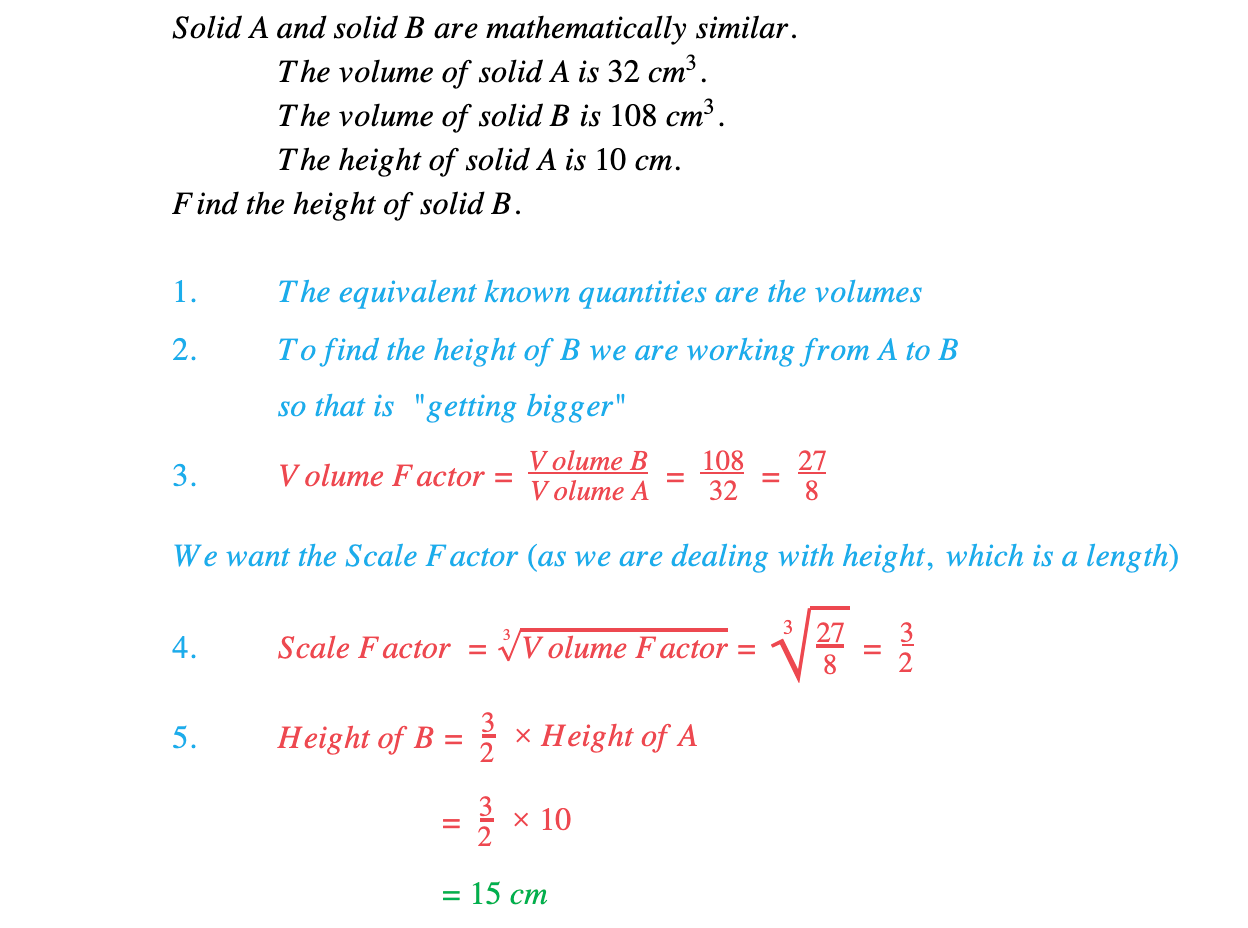# Edexcel IGCSE Maths 复习笔记 4.18.1 Similarity - Areas & Volumes

Edexcel IGCSE Maths 复习笔记 4.18.1 Similarity - Areas & Volumes

#### What are similar shapes?

• See Similarity – Lengths and know that:
• Equivalent areas are linked by an area factor (see below)
• Equivalent volumes are linked by a volume factor (see below)

#### Working with similar shapes

1. Identify equivalent known quantities (Lengths, Areas or Volumes)
2. Establish direction (getting bigger or smaller?)
3. Find Scale/Area/Volume Factor = Second Quantity ÷ First Quantity(Check Factor > 1 if getting bigger and Factor < 1 if getting smaller)
4. Convert between Scale/Area/Volume Factors usingArea Factor = (Scale Factor)2 ( Scale Factor = √(Area Factor) )Volume Factor = (Scale Factor)3 ( Scale Factor = ∛(Volume Factor) )
5. Use Scale/Area/Volume Factor to find new quantity

#### Worked Example